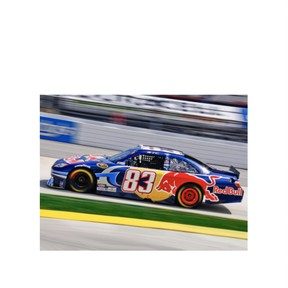Kinetic energy

# Kinetic energy

I can explain how the velocity and mass of an object affect its kinetic energy.8,000 schools use Gynzy92,000 teachers use Gynzy1,600,000 students use Gynzy

## General

In this lesson, students will learn the formula and definition of kinetic energy. They will then use that formula to compare the kinetic energy in various situations. They will also learn an overview of energy in general.

NGSS: MS-PS3-1

## Learning objective

Students will be able to explain how the velocity and mass of an object affect its kinetic energy.

## Introduction

Students will warm up with a scenario about building something out of wood. They will write the steps and the energy required for the process. They will discuss the work being done in this scenario.

## Instruction

The definition of energy, work, and kinetic energy will be introduced. Students will be presented with different scenarios, such as playing tennis and moving cars, and discuss the energy transfer in each scenario. They will also learn the formula for kinetic energy and how to find the kinetic energy of an object.

## Quiz

Review concepts about kinetic energy with 4 true/false questions and 6 multiple-choice questions.

## Closing

Students will discuss real-life examples where they see kinetic energy. Students will complete an exit slip with two short-answer questions about kinetic energy.

### The online teaching platform for interactive whiteboards and displays in schools

• Save time building lessons

• Manage the classroom more efficiently

• Increase student engagement